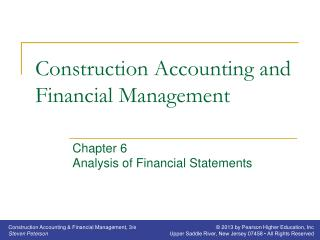# Construction Accounting and Financial Management - PowerPoint PPT PresentationDownload PresentationConstruction Accounting and Financial Management

Construction Accounting and Financial ManagementDownload Presentation## Construction Accounting and Financial Management

- - - - - - - - - - - - - - - - - - - - - - - - - - - E N D - - - - - - - - - - - - - - - - - - - - - - - - - - -
##### Presentation Transcript

1. Construction Accounting and Financial Management Chapter 6Analysis of Financial Statements

2. Financial Ratios • Affected by: • Method of depreciation • Retention • Timing of financial statements • When comparing items on the balance sheet and income statement, use the average of the balance before and after the period covered by the income statement

3. Quick Ratio • Ability to pay current (short-term) liabilities with cash or other near cash assets • Quick Ratio = (Cash + Accounts Receivable) Current Liabilities • Accounts receivable-retention should not be included in the accounts receivable • Ideal is 1.00 to 1

4. Current Ratio • Ability to use current assets to pay for current liabilities • Current Ratio = Current Assets Current Liabilities • Ideal is 2.00 to 1

5. Current Liabilities to Net Worth Ratio • Measurement of the risk that short-term creditors are taking by extending credit • CL to NW = Current Liabilities/Net Worth • Ideal is 67% for other industries • Construct exceeds this because of heavy use of trade financing

6. Debt to Equity Ratio • Risk in the company all creditors are taking compared to the risk the company’s owners are taking • Debt to Equity = Total Liabilities/Net Worth • Ideal is less than 2.00 to 1

7. Fixed Assets to Net Worth Ratio • Measurement of the amount of the owner’s equity that is tied up in fixed assets • FA to NW = Net Fixed Assets/Net Worth

8. Current Assets to Total Asset Ratio • Measurement of how liquidity a construction company’s assets are • CA to TA = Current Assets/Total Assets • Ideal is: • 0.55 to 0.65 for equipment intensive areas • 0.70 to 0.80 for all others

9. Collection Period • Measurement of the average time it takes a company to collect its accounts receivable • Exclude accounts receivable-retention • Measurment of how long the company’s capital is being used to finance client’s construction projects • Include accounts receivable-retention

10. Collection Period • Coll. Period = Accounts Receivable(365) Revenues • Ideal is less than 45 days

11. Average Age of Accounts Payable • Measure of how extensively a company is using trade financing • AA of AP = Accounts Payable(365) (Materials + Subcontract) • Assumes the bulk of the invoices that pass through the accounts payable are material and subcontract construction costs • Ideal is less than 45 days

12. Assets to Revenues Ratio • Measurement of how efficiently the company is using its assets • Assets to Revenues = Total Assets Revenues

13. Working Capital Turns • Measurement of how efficiently a company is using its working capital • Working capital: • The working capital represents those funds available for future operations or for the reduction of long-term liabilities • WC = Current Assets – Current Liabilities

14. Working Capital Turns • WCT = Revenues/Working Capital • When payments pass through to subcontractors: • WCT = (Revenues – Subcontractor) Working Capital

15. Accounts Payable to Revenue Ratio • Measurement of how much a company is using its suppliers and subcontractors as a source of funds • AP to R = Accounts Payable/Revenue • Includes accounts payable-retention

16. Gross Profit Margin • Percentage of the revenues left after paying construction costs and equipment costs • Measure of what percentage of each dollar of revenue is available to cover general overhead expenses and provide the company with a profit • Gross Profit Margin = Gross Profit/Revenue

17. General Overhead Ratio • Percentage of the revenues used to pay the general overhead expense • General Overhead = General Overhead Revenue • Ideal is less than 10% plus realtor fees

18. Profit Margin • Percentage of the revenues that becomes profit • Pretax PM = Net Profit Before Taxes Revenues • Ideal is >5% • After-tax PM = Net Profit After Taxes Revenues

19. Return on Assets • Measurement of how efficiently a construction company is using its assets • Return on Assets = Net Profit After Taxes Total Assets

20. Return on Equity • Return the company’s shareholders received on their invested capital • Pretax ROE = Net Profit Before Taxes Equity • Ideal is >15% • After-tax ROE = Net Profit After Taxes Equity

21. Degree of Fixed Asset Newness • Measurement of how new a company’s assets are • Affected by depreciation method • D of FAN = Net Fixed Assets Total Fixed Assets • Ideal is between 60 and 40%,

22. Months in Backlog • Measurement of work on hand • Affected by depreciation method • Mo in Backlog = Uncomp Work on Hand x 12 Revenues for 12 mo